• Engineering and Architecture
• Medicine and Allied Sciences
• Law
• Animation and Design
• Media, Mass Communication and Journalism
• Finance & Accounts
• Computer Application and IT
• Pharmacy
• Hospitality and Tourism
• Competition
• School
• Arts, Commerce & Sciences
• Learn
• Online Courses and Certifications

# Class 11 Maths: Cricket, Parabolas And Algebra Walk Into The Field

###### By Vipul Sachdeva###### Synopsis

Is it possible to predict whether a ball once bowled will hit the stumps based on the path it has followed? It is and that is what the Hawk Eye does. Applying mathematics, the “computer vision system” can assist the umpire with decision-making. Using this, the concept of parabolas in Class 11 Maths can be explained. It also helps to illustrate the relationship between graphs and equations and makes it easier to visualise problems that otherwise seem complex###### Synopsis

Is it possible to predict whether a ball once bowled will hit the stumps based on the path it has followed? It is and that is what the Hawk Eye does. Applying mathematics, the “computer vision system” can assist the umpire with decision-making. Using this, the concept of parabolas in Class 11 Maths can be explained. It also helps to illustrate the relationship between graphs and equations and makes it easier to visualise problems that otherwise seem complex

Indians love Cricket. It is even considered a religion by many. If you are a fan of the game, you must know what “Hawk Eye” is. It is a technology that helps umpires predict the path of the ball. Mostly used in the case of Leg Before Wicket (LBW) decisions, it tracks the ball’s path till the point of impact using cameras, and then extrapolates it to the stumps. If the ball is found to be hitting the stumps, below the height of the stumps and in front of them, the batsman is declared out.

Let us understand this process with the help of a mathematical model, for the Hawk Eye’s process is an example of applied mathematics. Let us first quickly recall how to make a straight-line graph.

If we are given the equation of straight line y = x + 1, we can make its graph by putting different values of x in the formula and obtaining corresponding values of y. For example, if we put x=1 in the equation, we get y = 2. So these values of x and y, when put in an ordered pair (1,2), will give us one point (say A) whose coordinates are (1,2) and which lies on the graph. We can similarly put x=2, and obtain y = 3. So, this point B (2,3) also lies on this graph. In the same way, we can obtain more points like C(3,4), D(4,5), etc. Now we can plot these points on the graph paper and join them to obtain the graph of y = x + 1. The graph will be as shown below.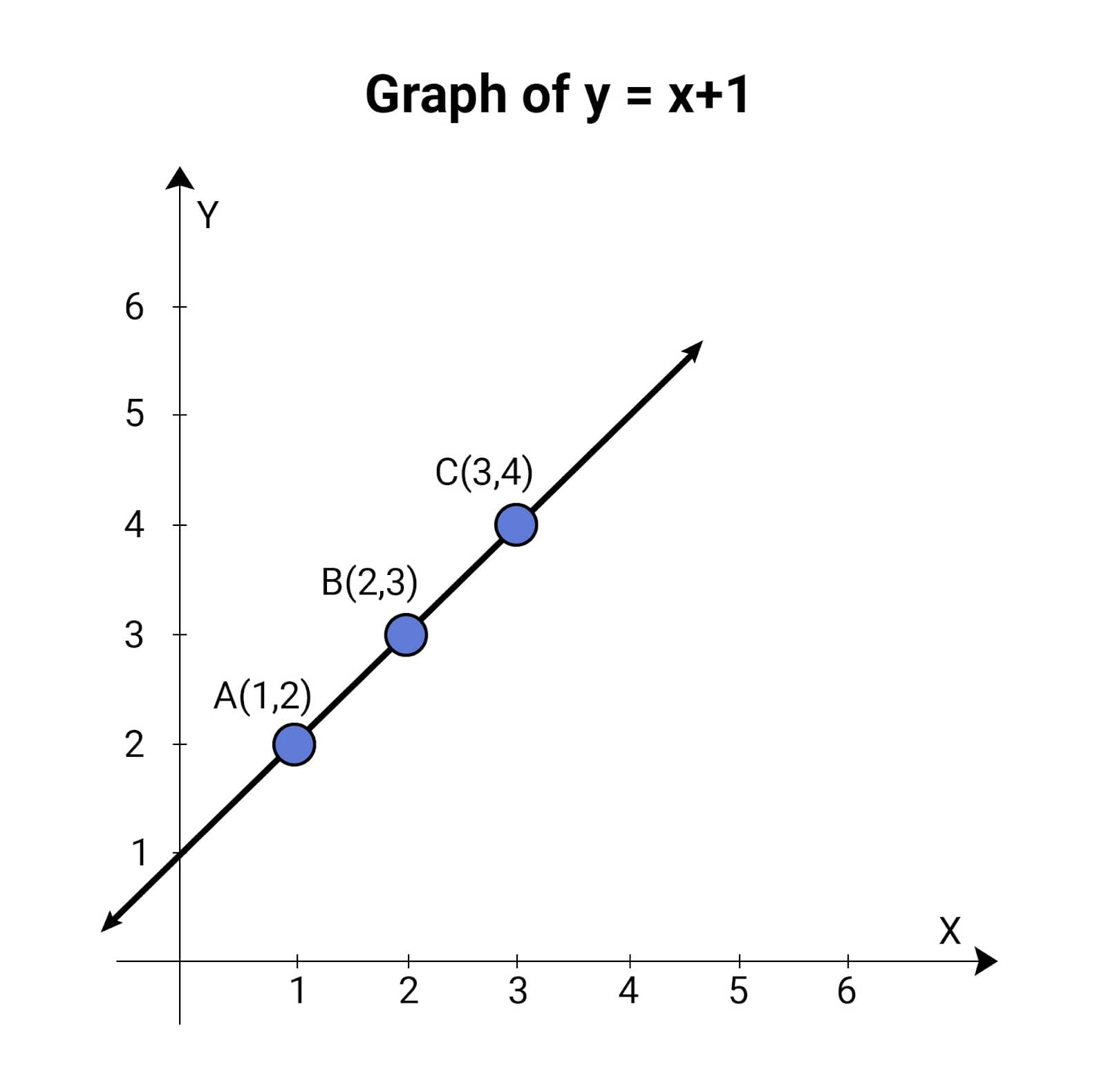Graph of y=x+1

Now, if we are given the graph, we can also find its equation. If the graph is as shown below, we can select any two points on it, say A (1,3) and B (2,4), and deduce that the equation is y = x +2.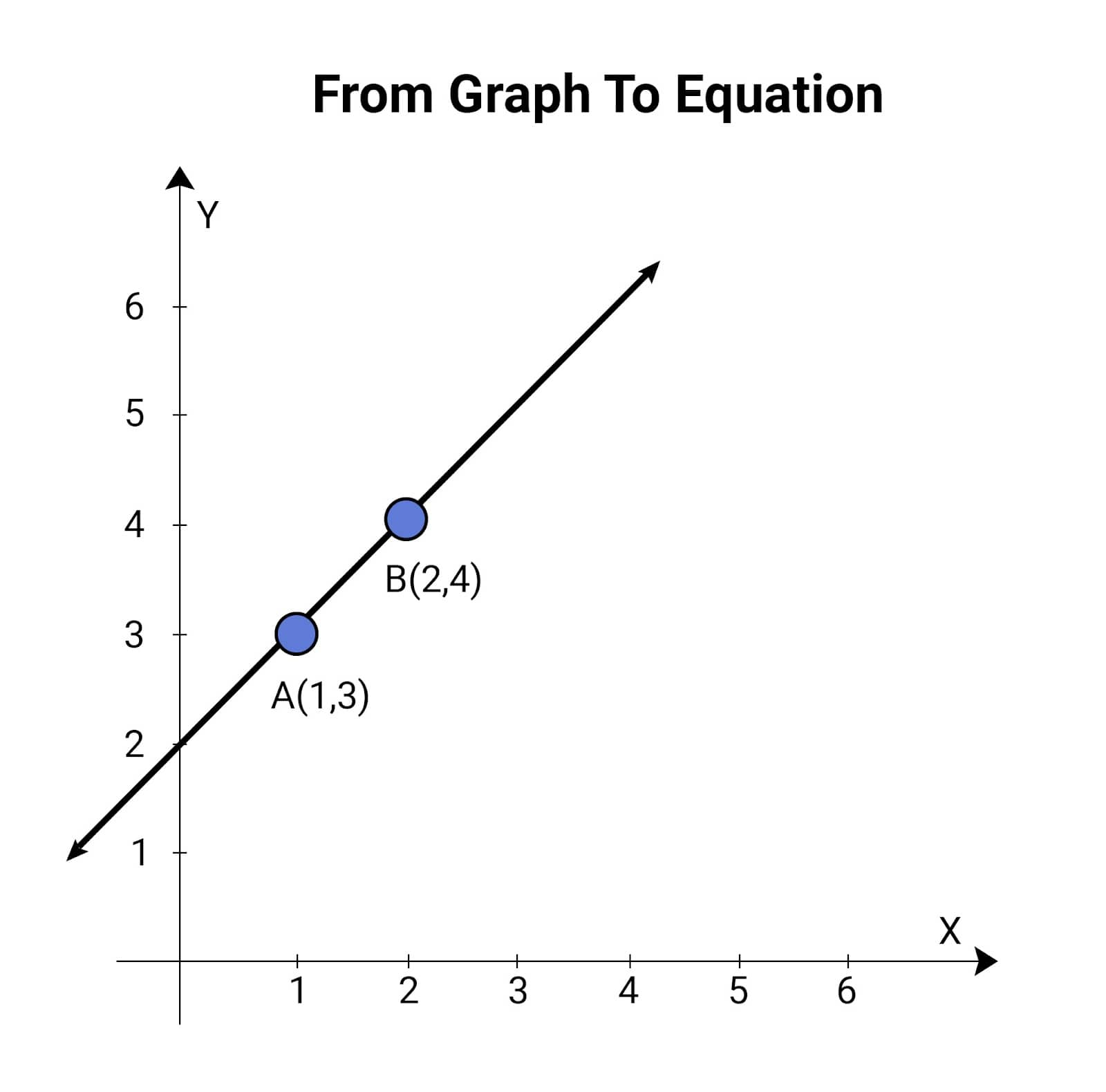From graph to equation

We also have the method of solving two linear equations to get the exact equation for a complex case but we won’t address that here. The important point to note is that we can make a graph from an equation, and we can also find the equation from the graph by using some computations.

## Cricket And Class 11 Maths

Now, we come back to cricket. When the ball is bowled, the path it follows is a parabola. Parabola is a curve which can be represented by the general expression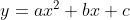where a, b, c are constants, which are different for different parabolas (this is a simplified form of parabola). So, if a computer can plot the path of the ball on graph paper, and compute the equation from the graph, it can predict whether the ball will hit the stump or not.

The following exercise will make it clearer. Say, after the ball bounces off the pitch, computers plot the path of the ball till it hits the batsman’s pads. This path will be a parabola. If the equation of the path calculated by the computer is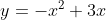can we tell conclusively if the ball hit the stumps? Let’s find out.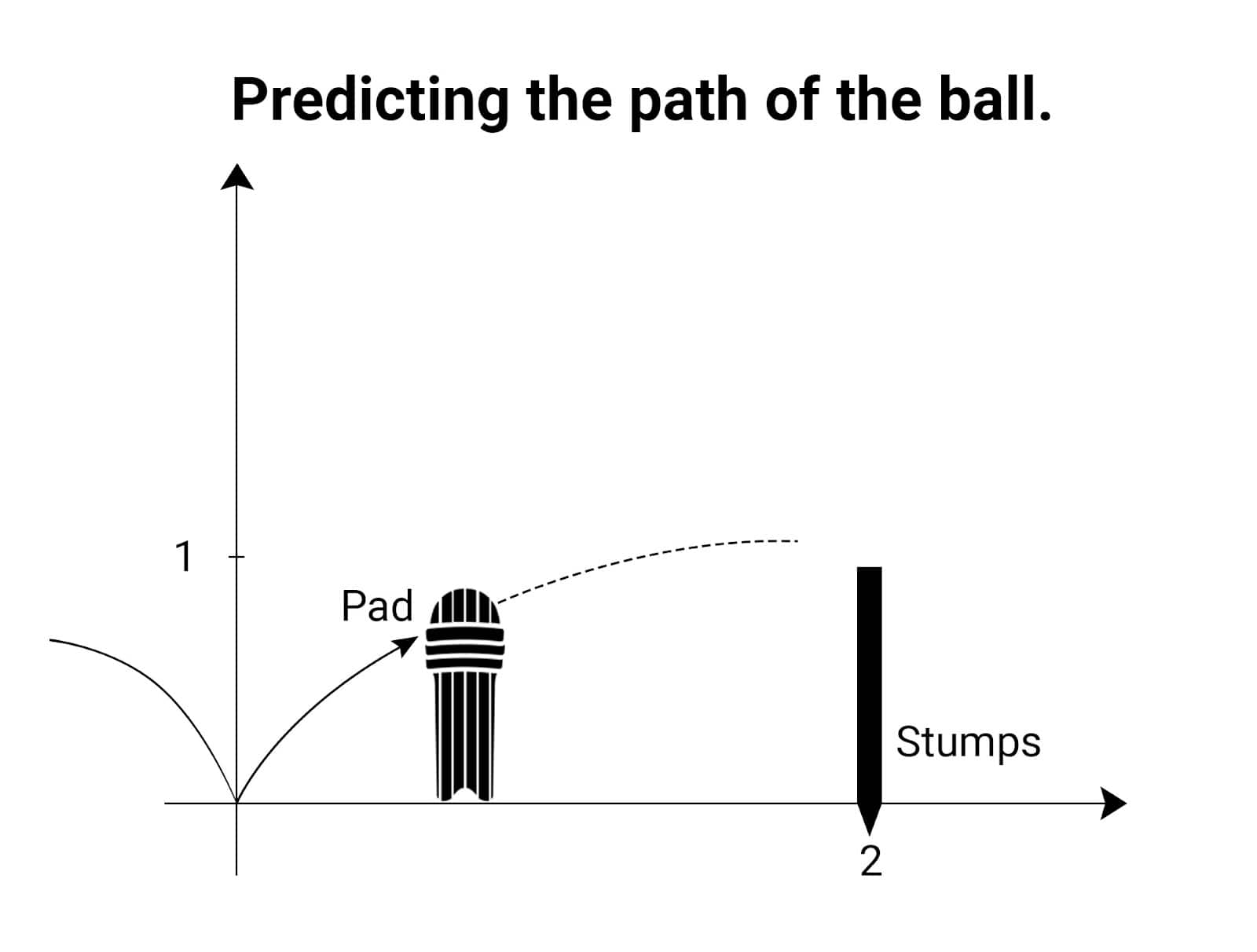Predicting the path of the ball

As it is clear from the graph, the value of x at the stumps is x = 2, and the height of the stumps is 1 (units are in meters). Let us find the height (y coordinate) of the ball, when it reaches the stumps. Putting x = 2 in the equation,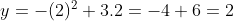meters. Clearly, the ball’s height is more than the stumps, and it will not hit the stumps, and thus, the batsman is not out.

We can similarly have different instances with different equations and check whether the ball would have hit the stumps or not. Now, let us make you the umpire and let you find out whether a batsman is out or not for the following scenarios:

1. The stumps are at x = 3, and the equation of the line after pitching is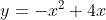The height of the stumps is 1 meter.

1. The stumps are at x = 5, and the equation of the line after pitching is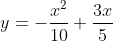The height of the stumps is again 1 meter.

Through these exercises, we hope you learned about the relationship between graphs and equations. In your studies, you should try to think of different functions in terms of graphs. That makes some problems, which otherwise look very complex using pure algebra, much easier to visualize and solve.

• Mathematics
• Mathematical objects
• Equation
• Parabola
• Batting (cricket)
• Cricket
• S
• p

## Subscribe to Premium Membership Plan

##### *Unlock all premium content and benefits:
• Career Guidance

Plan ahead with clear directions on courses, careers and best ways to learn

• Fees & Scholarships

• Jobs & Internships

Prepare for employed life with tips on jobs, find paid & unpaid internship listings, summer jobs and career advice.

• Interactive Counselling Webinars

• College Placement Reports

Wondering what comes after college? Read placement reports of top universities, colleges.

• Sports and Extra Curricular Activities

Interested in music? Art? Summer camps? Start exploring here.

• Mental Health & Well Being

To help you deal with stress, face exams, stay focussed, experts share tips here.

• Career Foundation

It's not too early to train for work life. Read about building CVs, cracking job interviews; find paid & unpaid internship listings, summer jobs and career advice.

• Study Help

Understand concepts of Physics, Chemistry Maths and others through fun stories and illustrations

• NCERT, Exemplar, CBSE Previous year Solutions for Maths & Science

- Concept-oriented detailed solutions for NCERT (Class 6 to 12), Exemplar (Class 9 to 12) & CBSE Previous 5 year (Class 10 and 12)

- For Maths and Science

• 15+ B.Tech College Predictors

Analyses your exam performace to predict admission chances based on Domicile, Caste, Gender, etc. and Exam Result.

Get your personalised report with Top Predicted Colleges.

• NCHMJEE Hospitality College Predictor

- Get your NCHM JEE personalised report with Top Predicted IHMs, Government & Private Colleges delivered via email

• NIFT Design College Predictor

- Know your admission chances based on your Home State, Caste, etc. & exam result for NIFT campuses

- Get your NIFT personalised report for B.Des & B.FTech delivered via email

• CLAT, AILET, DUET L.L.B, MHCET Law College Predictors

- Know your admission chances in NLUs, Delhi University’s Faculty of Law for L.L.B (3Yr), National Law University, Delhi’s B.A-L.L.B course & Law colleges accross Maharashtra based on your Seat Type, Caste, etc. & exam results

- Get your personalised report delivered via email

Interactive webinars with experts for different countries will take you through all the basics.

• SOP Guidance

What should go into your statement of purpose (SOP)? Learn how to put together a winning application.

• English Proficiency Test

How can you ace the English proficiency test? Let the experts help you.

• Visa Regulations

• Career Support

Exams and scholarship alerts, test prep reviews, study plans, college selection

## Rate this Story

• Poor
• Weak
• Good
• Very Good
• Excellent## Careers360 helping shape your Career for a better tomorrow#### 250M+

Students#### 30,000+

Colleges#### 500+

ExamsE-Books